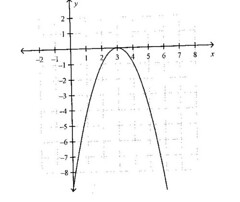5 Questions | Total Attempts: 353SettingsComplete the following questions

• 1.
Which correctly identifies the values of the parameters a, h, and k for the function f(x) = –2(x + 3)^2 + 1
• A.

A = –2, h = 3, k = 1

• B.

A = 2, h = –3, k =–1

• C.

A = –2, h = –3, k = 1

• D.

A = –2, h = –3, k = –1

• 2.
What is the equation of this graph
• A.

Y = –x^2 + 3

• B.

Y = –3x^2

• C.

Y = –(x + 3)^2

• D.

Y = –(x – 3)^2

• 3.
Which function includes a translation of 3 units left?
• A.

F(x) = (x + 3)^2 + 1

• B.

F(x) = 3x^2 + 1

• C.

F(x) = (x – 3)^2 + 1

• D.

F(x) = (x + 1)^2 – 3

• 4.
Consider a parabola P that is congruent to y = x^2 and with vertex at (0, 0).  Find the equation of a new parabola that results if P is translated 3 units
• A.

Y = (x + 3)^2 + 4

• B.

Y = (x – 3)^2 + 4

• C.

Y = (x – 4)^2 + 3

• D.

Y = (x – 3)^2 – 4

• 5.
The formula for compound interest on \$1000at a given interest rate, r, is shown by the function f(r) = 1000(r + 1)^2.  What are the values of the parameters a, h, and k for f(r)
• A.

A = 1000, h = 1, k = 0

• B.

A = 1000, h = 0, k = 1

• C.

A = 0, h = 1000, k = 1

• D.

A = 1000, h = –1, k = 0

Related TopicsBack to top